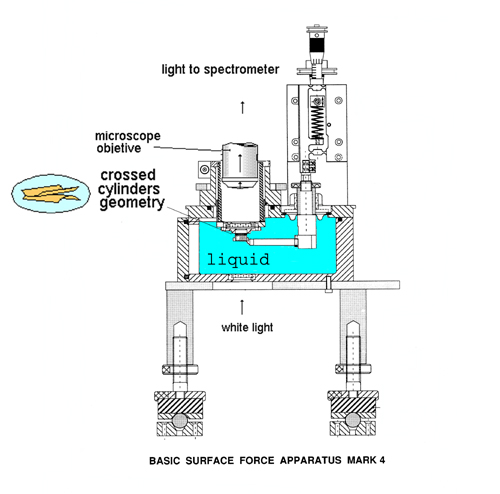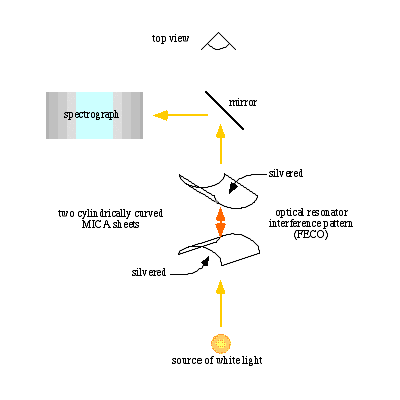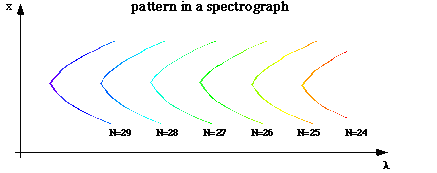Surface force apparatus measurements

The Surface Forces Apparatus, was developed at the Australian National University (Mk4 shown below), where J. Israelachvilli (now at UCSB) developed the SFA technique and early apparatus. The instrument is capable of measuring the forces between two molecularly smooth surfaces made of mica in vapors or liquids with a sensitivity of a few millidynes (10 nN) and a distance resolution of about 0.1nm. These flat smooth surfaces of mica can be covered to obtain the force between different materials.

The basic instrument, shown below, has a simple single-cantilever spring to which the lower silica disk is attached. This lower mica is brought near the upper mica by a piezoelectric device. If there is some interaction the distance between the micas will not be the same as that given by the piezoelectric device. Therefore, the force is measured by the gap distance difference given by the piezoelectric device and that measured directly by interferometry (attractive forces make the micas closer and repulsive forces try to move away the micas).Interferometry is used in the surface forces apparatus to measure the distance between the two surfaces of interest with high accuracy. Interferometry allows measuring distances down to 1/1000 of a wavelength. Multiple beam interferometry uses intense white light which is sent normally through the surfaces in the surface forces apparatus. Each surface (usually mica) has a highly reflecting silver coating on one side, therefore, both surfaces form an optical cavity. The white light is reflected multiple times on these mirrors before it leaves the interferometer, each time interfering with the previously reflected beams. Some particular wavelengths fit exactly an entire number of times inside the interferometer and lead to constructive interference.

The figure below shows a schematics of the multiple beam interferometrer at work in the instrument. A source of white light is focused between two silver-coated surfaces. The light emerging from the interferometer is sent to a spectrograph, and it consists of well defined wavelengths which fit exactly an entire number of times into the optical resonator in the form of curved fringes. The fringes are called Fringes of Equal Chromatic Order (FECO).Fringes of Equal Chromatic Order